## How to create a total average distinct count measure

Hi all

My task is going to build a bar chart with a a straight average line across the bars, could you send some lights on creating the average distinct count variable please? Currently the line is a trend line but I need a straight line cross the bars. Like the red line in below chart.

Variables:

Event ID - Distinct count (Event ID set as distinct count)

Event assigned QID upper northland (staff ID)

The formula and expected(in red line)/current(orange line) chart as below:

formula like sum the distinct count then divide by the distinct count staff id

`Sum [_ForAll_] (AggregateTable(_Sum_, Table(_Avg_, Fixed('EventNum-string'n, 'Event Assigned QIDs - upper northland'n),'Frequency'n))) / Distinct [_ByGroup_] ('Event Assigned QIDs - upper northland'n)`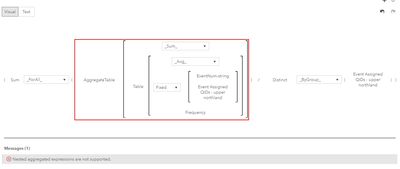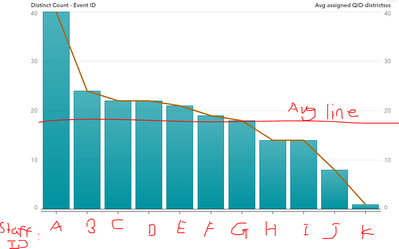Thanks

AC

1 ACCEPTED SOLUTION

Accepted Solutions

## Re: How to create a total average distinct count measure

It's tricky.

I would consider a two-steps approach with an aggregated table first. Then you can create an aggregated item on a fixed quantity preventing the error message you received.

Nevertheless you can achieve it, but it's so tricky that I recommend my earlier suggestion.

Here's the tricky solution:

The grand average distinct count in my opinion needs a higher aggregation to make it work. in my case this is year as the graph's x axis is month.

``````AggregateTable(_Sum_, Table(_Sum_, Fixed('_year'n), Table(
_CountDistinct_, Fixed('TMAIMG_MODELINFO'n, 'id'n), 1))) / 12``````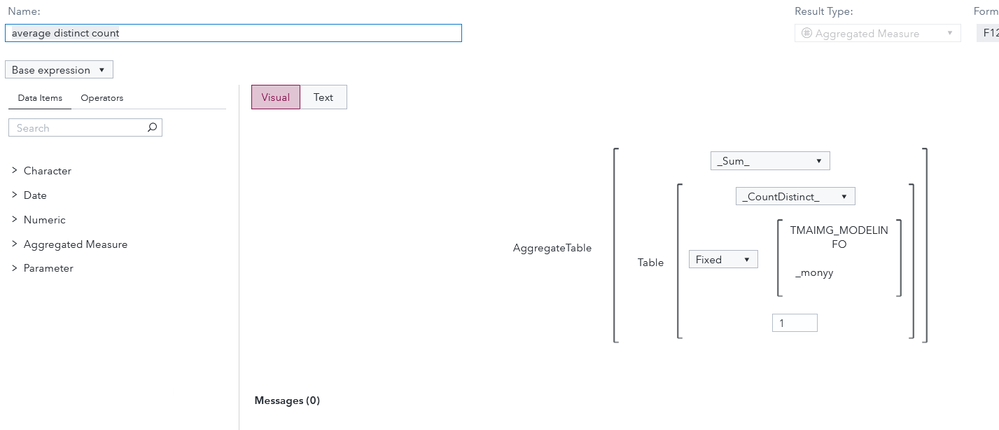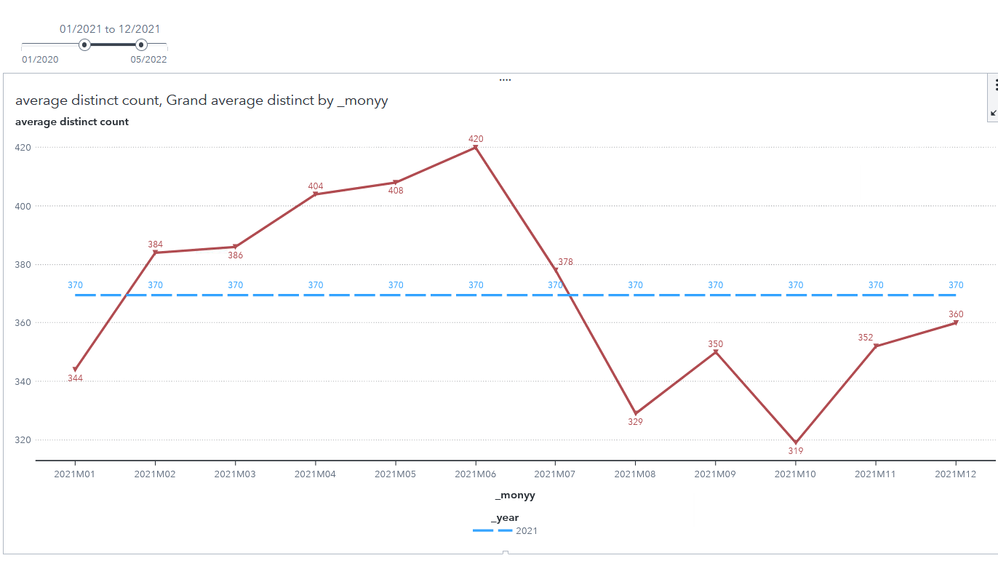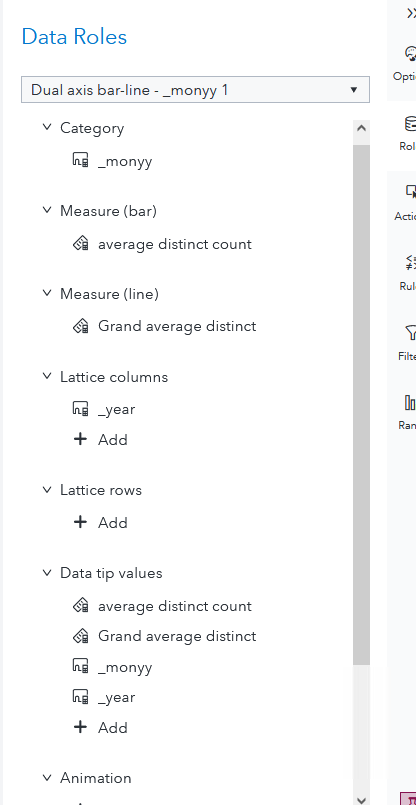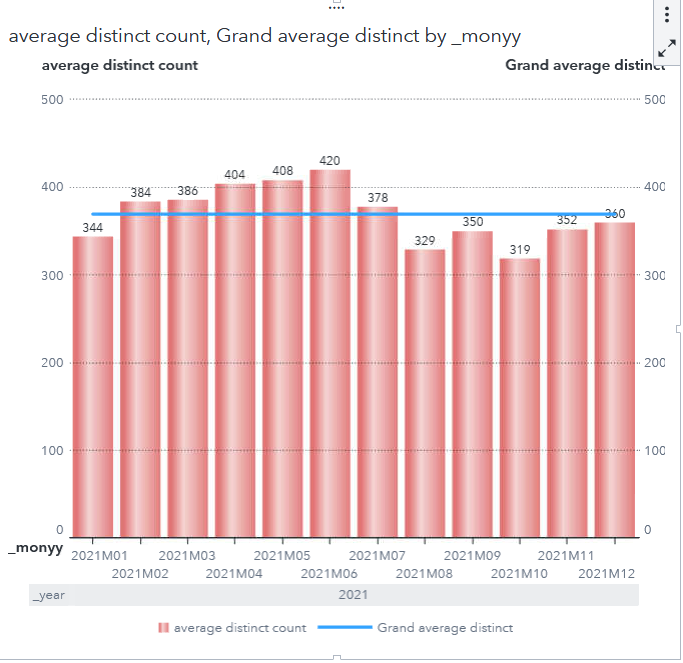## Re: How to create a total average distinct count measure

It's tricky.

I would consider a two-steps approach with an aggregated table first. Then you can create an aggregated item on a fixed quantity preventing the error message you received.

Nevertheless you can achieve it, but it's so tricky that I recommend my earlier suggestion.

Here's the tricky solution:

The grand average distinct count in my opinion needs a higher aggregation to make it work. in my case this is year as the graph's x axis is month.

``````AggregateTable(_Sum_, Table(_Sum_, Fixed('_year'n), Table(
_CountDistinct_, Fixed('TMAIMG_MODELINFO'n, 'id'n), 1))) / 12``````Discussion stats